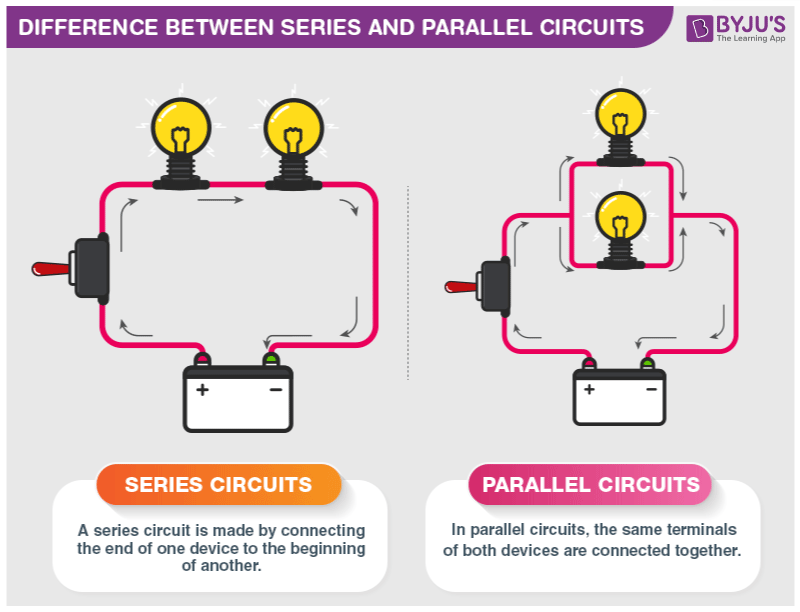# Difference between Series and Parallel Circuits

When there are two or more electrical devices in a circuit with an energy source, there are a couple of basic ways by which we connect them. They can either be connected in series or in parallel combinations. A series circuit is a circuit in which two components share a common node and the same current flows through them. However, in a parallel circuit, components share two common nodes. In this article, let us look at more differences between series connection and parallel connection circuits.

## What is a Series Circuit?

A circuit is said to be connected in series when the same current flows through all the components in the circuit. In such circuits, the current has only one path. Let us consider the household decorative string lights as an example of a series circuit. This is nothing but a series of multiple tiny bulbs connected in series. If one bulb fuses, all the bulbs in the series do not light up.

## What is a Parallel Circuit?

A circuit is said to be parallel when the electric current has multiple paths to flow through. The components that are a part of the parallel circuits will have a constant voltage across all ends.

## Difference Between Series and Parallel CircuitsThe major difference between series and the parallel circuit is the amount of current that flows through each of the components in the circuit. In a series circuit, the same amount of current flows through all the components placed in it. On the other hand, in parallel circuits, the components are placed in parallel with each other due to which the circuit splits the current flow. The current flowing from the source will be divided into the current flowing through each of these components.

Difference Between Series and Parallel Circuits
Series Parallel
The same amount of current flows through all the components The current flowing through each component combines to form the current flow through the source.
In an electrical circuit, components are arranged in a line In an electrical circuit, components are arranged parallel to each other
When resistors are put in a series circuit, the voltage across each resistor is different even though the current flow is the same through all of them. When resistors are put in a parallel circuit, the voltage across each of the resistors is the same. Even the polarities are the same
If one component breaks down, the whole circuit will burn out. Other components will function even if one component breaks down, each has its own independent circuit
If Vt is the total voltage then it is equal to V1 + V2 +V3 If Vt is the total voltage then it is equal to V1=V2=V3

## See the video below to learn about the Kirchoff’s law and combination of resistors in a detailed way## Frequently Asked Questions – FAQs

Q1

### In which type of circuit connection, the same amount of current flows through all the components?

Series Connection.

Q2

### What are the two types of circuit connections?

The two types of circuit connections are:

• Series connection
• Parallel connection
Q3

### What is a parallel circuit?

A circuit is said to be parallel when the electric current has multiple paths to flow through.

Q4

### How is the total resistance of any series circuit calculated?

Total resistance in a series circuit is equal to the sum of the individual resistances.
Q5

### What is the total resistance of a circuit, which has 2kΩ, 3kΩ and 4kΩ resistance connected in series with each other?

Total Resistance(R) = 2kΩ + 3kΩ + 4kΩ = 9kΩ

## Watch the video and solve important questions in the chapter Electricity Class 10Stay tuned to BYJU’S and Fall in Love with Learning!

Test your knowledge on Series and parallel circuit differences

1. Super

2. super i was clear and easily understandable

3. does that mean that the current in the parallel combination is different?am I right?

• In a parallel circuit, the current is different in each branch. There might be more current in one branch and less current in another branch. Nonetheless, the total amount of current when added together is the same as the amount of current at points outside the branches.

4. Which circuit is better: series or parallel circuit?

• We need both types of circuits to power and control appliances, so neither is better than the other.
To help you better understand their importance, I have listed different applications of series and parallel circuit:
1) Parallel circuits are mostly used at our homes and office buildings. They are used so that every appliance that you purchase locally, matches with the correct voltage at every outlet of the house.
2) Offices make use of parallel circuits to power the appliance but series circuits control the power.
3) Fuses and circuit breakers are an example of series circuits controlling operating parallel circuits.
4) Our computers contain millions of tiny series and parallel circuits working together to keep it functioning.

5. Why do we take the reciprocal in the parallel combination of resistors to find total resistance? While in the series combination of resistor we simply add each resistance?